ocr图像处理 - CSDN

• 因为处理图像都很大很大，居然有11000*12000这种分辨率的，有90M大小，我也是醉了，绝大部分都是6000左右分辨率的图像，这种图像直接送到CTPN里的话，效果不是太好，太大了 而且效率感人，所以必须做一下预处理。...
说明：文字方向校正(fft方式和放射变换方式)参考了网上的代码，只做了少量修改
只针对医疗影像图像，自然场景下的另说
因为处理的图像都很大很大，居然有11000*12000这种分辨率的，有90M大小，我也是醉了，绝大部分都是6000左右分辨率的图像，这种图像直接送到CTPN里的话，效果不是太好，太大了 而且效率感人，所以必须做一下预处理。大部分的X光图像很简单，直接缩放送CTPN即可，而CT和MRI图像虽然一张上有很多小图像，但好在要么有虚线分割要么中间都会留有空白的地方，于是就可以利用直线检测和投影检测来把图片分割成若干小图像了。(吐槽一下之前老外写的代码，不管三七二十一把所有图像都是分成上下两部分，然后上下再各分成上下两部分，四个部分再分别循环他的N个算法，搞的整个系统70%以上的资源都在跑OCR，一张很简单的图片最低也要几分钟才能出结果，复杂一点的都是10几分钟 真想知道这是怎么过验收的！)

图像分割，思想很简单 有虚线的直接做直线检测，有空白的做X、Y轴的投影，都没有的就是X光图像了，直接把整张图像当做ROI送CTPN

def _img_split_with_hough(img, min=100, max=220):
"""
:param img: 读入的二值化图
:param min: 边缘检测阈值
:param max: 边缘检测阈值
:return: 水平和垂直线的坐标集合
"""
h = img.shape
w = img.shape
edges = cv2.Canny(img, min, max)
lines = cv2.HoughLinesP(edges, 1, np.pi / 180, 30, minLineLength=100, maxLineGap=10)
lines1 = lines[:, 0, :]
h_line = []
v_line = []
for x1, y1, x2, y2 in lines1[:]:
if y2 == y1:
flag = False
for element in h_line:
if abs(element - y1) < 10:
flag = True
break
if flag == False and abs(x1 - x2) > w * 0.5:
h_line.append((x1, y1, x2, y2))
elif x1 == x2:
flag = False
for element in v_line:
if abs(element - x1) < 10:
flag = True
break
if flag == False and abs(y1 - y2) > h * 0.5:
v_line.append((x1, y1, x2, y2))
return h_line, v_line

"""
:param binary_img: 读入的灰度图
:param img_show:
:return: 水平和垂直线的坐标集合
"""
h = gray_img.shape
w = gray_img.shape

# 按行求和
sum_x = np.sum(gray_img, axis=1)
# 按列求和
sum_y = np.sum(gray_img, axis=0)

h_line_index = np.argwhere(sum_x < 10)
v_line_index = np.argwhere(sum_y < 10)

h_line_index = np.reshape(h_line_index, (h_line_index.shape,))
v_line_index = np.reshape(v_line_index, (v_line_index.shape,))

h_line = [(0, h_line_index, w - 1, h_line_index), (0, h_line_index[-1], w - 1, h_line_index[-1])] if len(
h_line_index) > 0 else []
v_line = [(v_line_index, 0, v_line_index, h - 1), (v_line_index[-1], 0, v_line_index[-1], h - 1)] if len(
v_line_index) > 0 else []

for i in range(len(h_line_index) - 1):
if h_line_index[i + 1] - h_line_index[i] > 2:
h_line.append((0, h_line_index[i], w - 1, h_line_index[i]))

for i in range(len(v_line_index) - 1):
if v_line_index[i + 1] - v_line_index[i] > 2:
v_line.append((v_line_index[i], 0, v_line_index[i], h - 1))

return h_line, v_line

def _combine_rect(h_lines, v_lines, w, h):
rects = []
# 添加第一行(列)和最后一行(列)
x_axis = sorted(set([0, w - 1] + [item for item in v_lines]))
y_axis = sorted(set([0, h - 1] + [item for item in h_lines]))

point_list = []
for y in y_axis:
point = []
for x in x_axis:
point.append((y, x))
point_list.append(point)

for y_index in range(len(y_axis) - 1):
if y_axis[y_index + 1] - y_axis[y_index] <= 10:
continue
for x_index in range(len(x_axis) - 1):
if x_axis[x_index + 1] - x_axis[x_index] <= 10:
continue
rects.append((y_axis[y_index], x_axis[x_index],
y_axis[y_index + 1], x_axis[x_index + 1]))
return rects

def img_split(img_file, threshold_value=180, img_show=False):
"""

:param img_file: 输入图片路径
:param img_show: 是否显示
:return: 分割后的子图像rect列表
"""
gray = cv2.cvtColor(img, cv2.COLOR_BGR2GRAY)
gray = color_nomal(gray)
# ret, binary = cv2.threshold(gray, threshold_value, 255, cv2.THRESH_BINARY)
h = img.shape
w = img.shape
rate = h // w if h > w else w // h

if len(h_line) < 1 and len(v_line) < 1:
h_line, v_line = _img_split_with_hough(gray)

rects = _combine_rect(h_line, v_line, w, h)
split_imgs = []
for rect in rects:
split_imgs.append(img[rect:rect, rect:rect])

if img_show:
for rect in rects:
cv2.rectangle(img, (rect, rect), (rect, rect), (0, 255, 0), 2)
img = cv2.resize(img, (int(h * 0.7), int(h * 0.7 / rate)))
cv2.imshow('cece', img)
cv2.waitKey()
return split_imgs

分割结果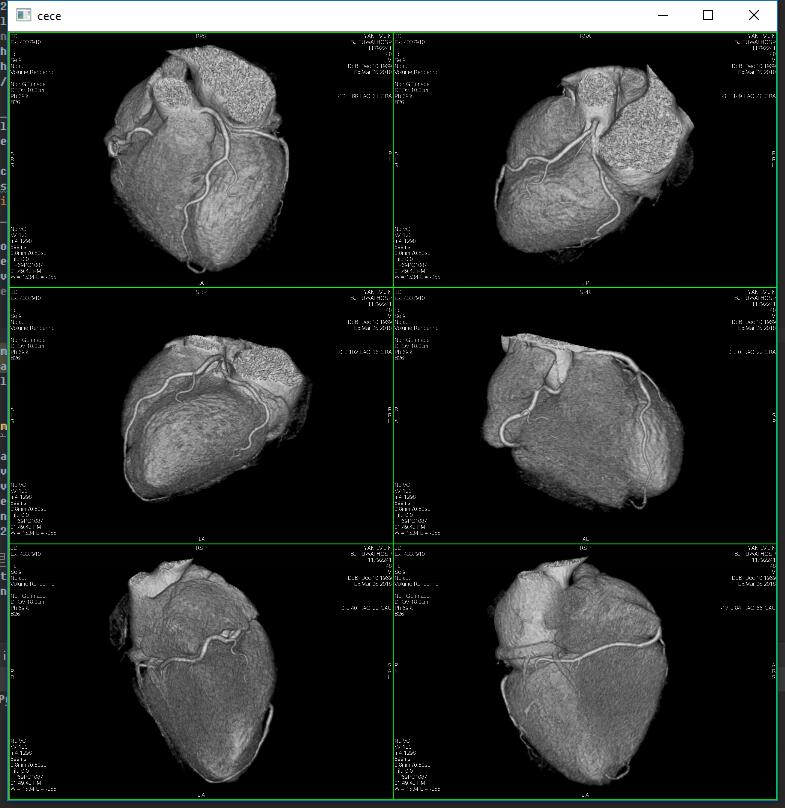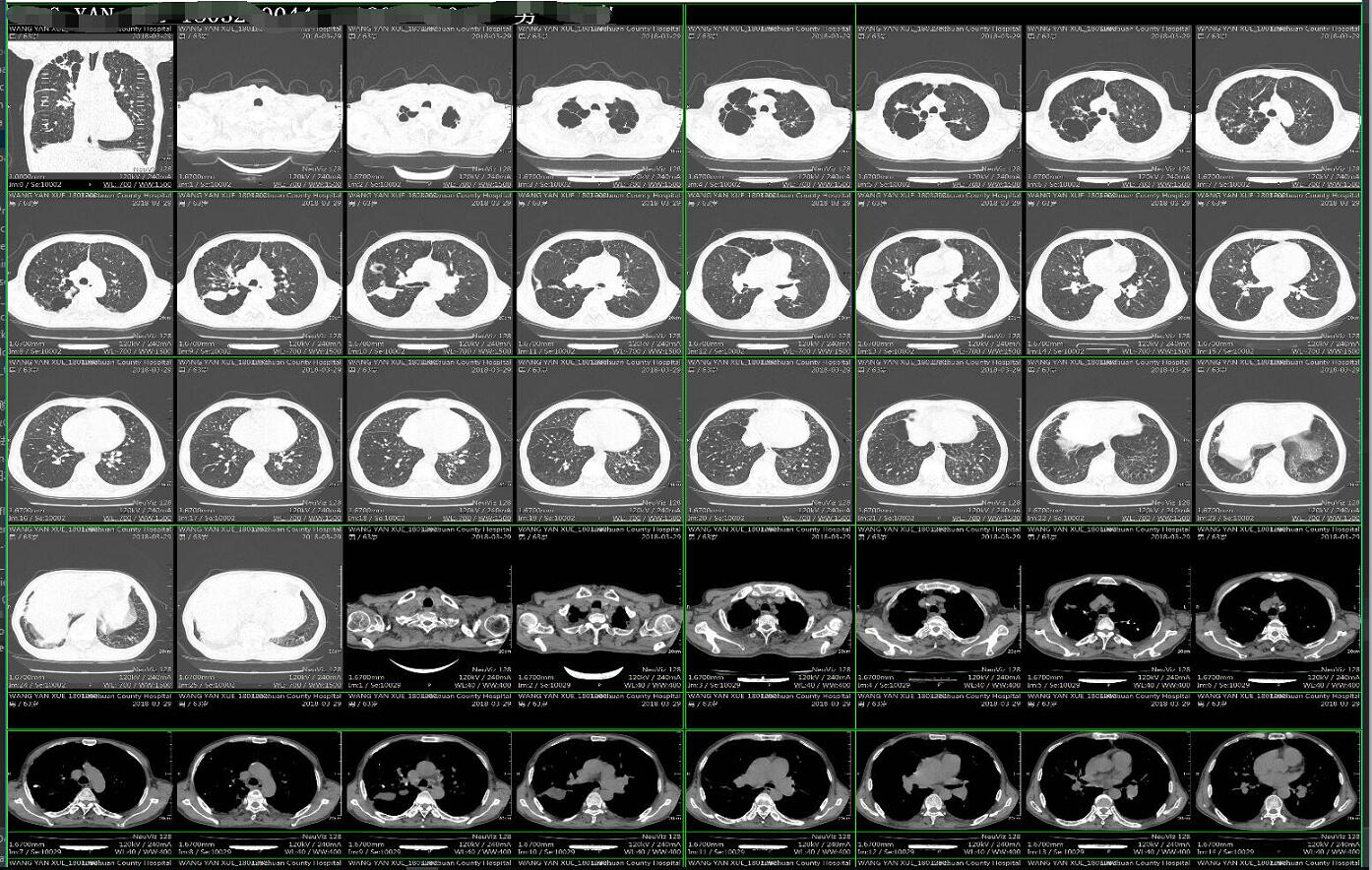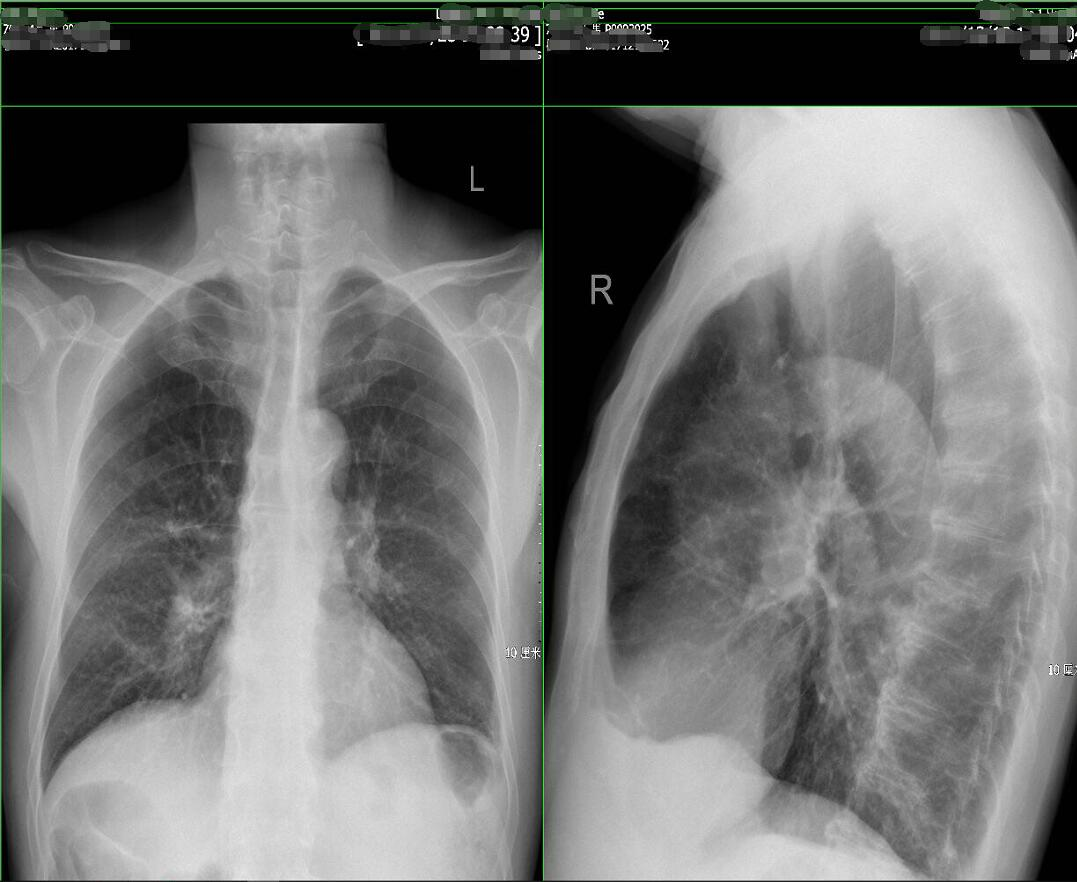2. 文字方向校正，可以使用FFT变换后校正然后再逆变换回来，也可以直接使用查找包含文字区域的矩形，旋转这个矩形，但是这种方法对于垂直的图像就没效果了，因为会发现包含文字的矩形区域就是方方正正的 不用校正。在二值化的时候采用了自适应二值化，这样做的好处是能更精确的定位文字区域，全局二值化可能会造成有些地方一团黑。
def rotated_img_with_fft(gray):
# 图像延扩
h, w = gray.shape[:2]
new_h = cv2.getOptimalDFTSize(h)
new_w = cv2.getOptimalDFTSize(w)
right = new_w - w
bottom = new_h - h
nimg = cv2.copyMakeBorder(gray, 0, bottom, 0, right, borderType=cv2.BORDER_CONSTANT, value=0)

# 执行傅里叶变换，并过得频域图像
f = np.fft.fft2(nimg)
fshift = np.fft.fftshift(f)

fft_img = np.log(np.abs(fshift))
fft_img = (fft_img - np.amin(fft_img)) / (np.amax(fft_img) - np.amin(fft_img))

fft_img *= 255
ret, thresh = cv2.threshold(fft_img, 150, 255, cv2.THRESH_BINARY)

# 霍夫直线变换
thresh = thresh.astype(np.uint8)
lines = cv2.HoughLinesP(thresh, 1, np.pi / 180, 30, minLineLength=40, maxLineGap=100)
try:
lines1 = lines[:, 0, :]
except Exception as e:
lines1 = []

# 创建一个新图像，标注直线
# lineimg = np.ones(nimg.shape,dtype=np.uint8)
# lineimg = lineimg * 255

piThresh = np.pi / 180
pi2 = np.pi / 2
angle = 0
for line in lines1:
# x1, y1, x2, y2 = line
x1, y1, x2, y2 = line
# cv2.line(lineimg, (x1, y1), (x2, y2), (0, 255, 0), 2)
if x2 - x1 == 0:
continue
else:
theta = (y2 - y1) / (x2 - x1)
if abs(theta) < piThresh or abs(theta - pi2) < piThresh:
continue
else:
angle = abs(theta)
break

angle = math.atan(angle)
angle = angle * (180 / np.pi)
print(angle)
# cv2.imshow("line image", lineimg)
center = (w // 2, h // 2)
M = cv2.getRotationMatrix2D(center, angle, 1.0)
M[0, 2] += (width_1 - w) / 2
M[1, 2] += (height_1 - h) / 2
rotated = cv2.warpAffine(gray, M, (width_1, height_1), flags=cv2.INTER_CUBIC, borderMode=cv2.BORDER_REPLICATE)
cv2.imshow('rotated', rotated)
cv2.waitKey(0)
return rotated

if is_show:
cv2.imshow('thresh', thresh)
# 计算包含了旋转文本的最小边框
coords = np.column_stack(np.where(thresh > 0))

# 该函数给出包含着整个文字区域矩形边框，这个边框的旋转角度和图中文本的旋转角度一致
angle = cv2.minAreaRect(coords)[-1]
print(angle)
# 调整角度
if angle < -45:
angle = -(90 + angle)
else:
angle = -angle
# 仿射变换
h, w = gray.shape[:2]
center = (w // 2, h // 2)
print(angle)
M = cv2.getRotationMatrix2D(center, angle, 1.0)
rotated = cv2.warpAffine(gray, M, (w, h), flags=cv2.INTER_CUBIC, borderMode=cv2.BORDER_REPLICATE)
if is_show:
cv2.putText(rotated, 'Angle: {:.2f} degrees'.format(angle), (10, 30), cv2.FONT_HERSHEY_SIMPLEX, 0.7,
(0, 0, 255), 2)
print('[INFO] angel :{:.3f}'.format(angle))
cv2.imshow('Rotated', rotated)
cv2.waitKey()
return rotated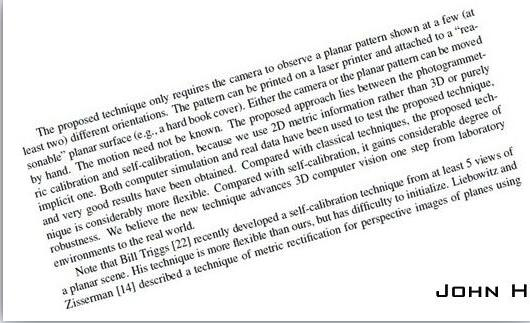放射校正结果：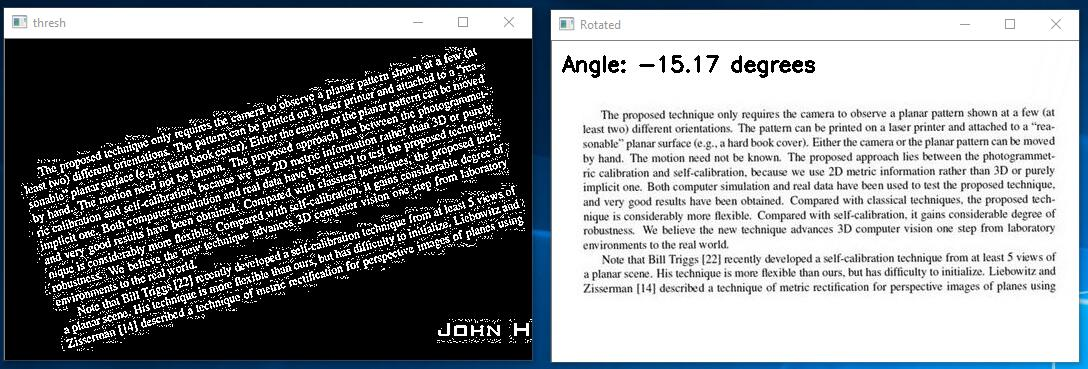原图：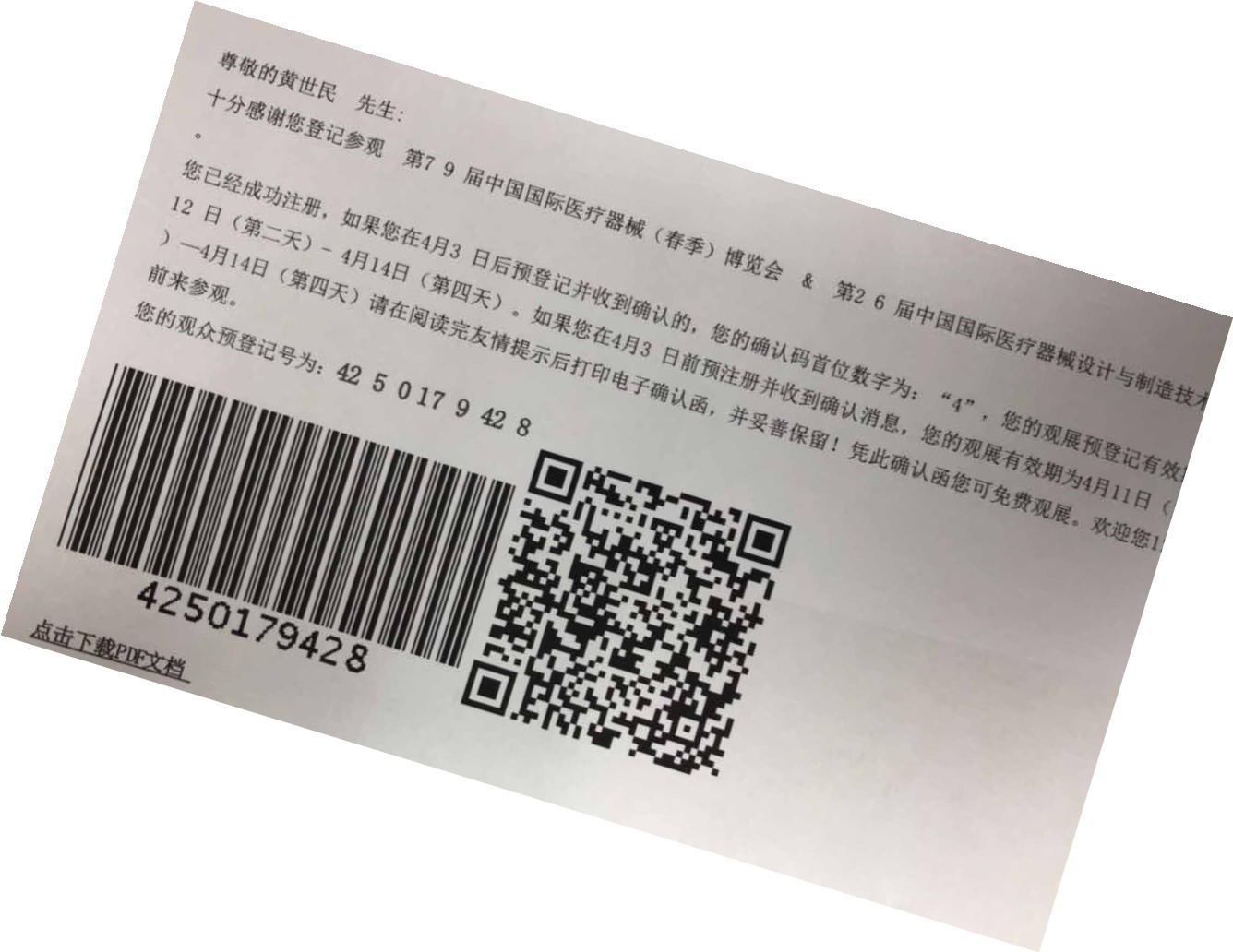放射校正：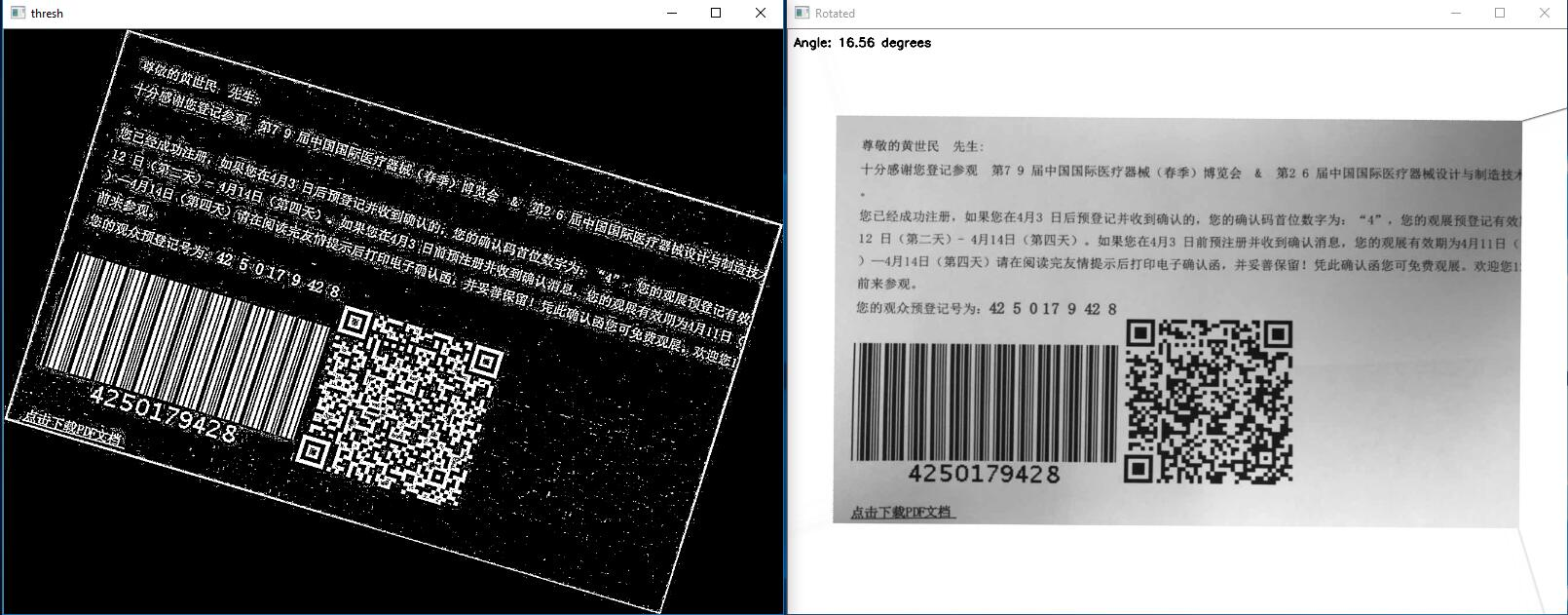fft校正，计算的时候有大概2度的误差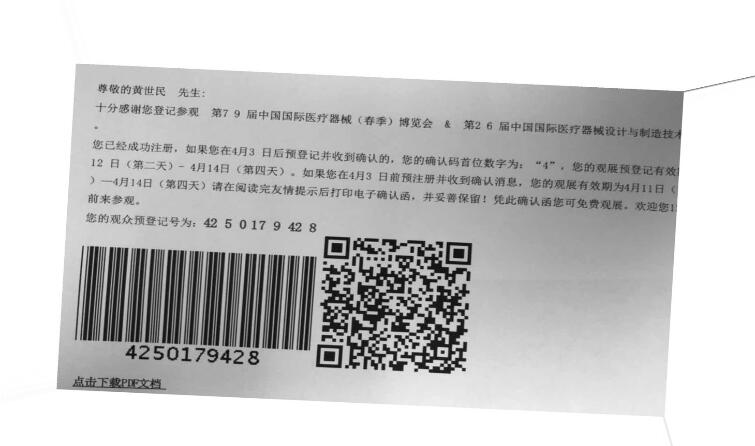展开全文图像分割
• 仿射 affine 平面内的内移，旋转，错切，缩放，变换后仍在一个平面 三个点 仿射变换：向量经过线性变换+平移 6个未知数，6个方程，1个点列2个方程，需要3个点坐标 投影 projective ...
仿射 affine
平面内的内移，旋转，错切，缩放，变换后仍在一个平面
三个点
仿射变换：向量经过线性变换+平移

6个未知数，6个方程，1个点列2个方程，需要3个点坐标

投影 projective
物体与投影面上的像之间的变换，变换前后不在一个平面
四个点

12个未知数，12个方程，1个点列3个方程，需要4个点坐标

IOU分类中的交叉熵

字符串编辑距离（levenshtein距离）
计算两个字符串间的差异程度的字符串度量，可以认为是从字符串a修改到字符串b需要的单个字符编辑次数最小值
eg：kitten --> sitting levenshtein=3
k->s sitten
e->i sittin
_->g sitting

实现方法：动态规划
https://blog.csdn.net/ls386239766/article/details/38961745


展开全文• 1、文档图像角度倾斜矫正：1）霍夫直线检测，从直线方向的众数得到旋转角度；2）文字区域较小时可通过最小外接矩形得到旋转角度； 2、打印痕迹干扰，可使用双边滤波进行降噪，但不能完全去除； 3、图像退化，不...
1、文档图像角度倾斜矫正：1）霍夫直线检测，从直线方向的众数得到旋转角度；2）文字区域较小时可通过最小外接矩形得到旋转角度；

2、打印痕迹干扰，可使用双边滤波进行降噪，但不能完全去除；

3、图像退化，不清晰，可使用高反差得到文字轮廓信息，再与原图相加；

以上方法在tesseract里封装完成。降噪对于OCR非常重要。

展开全文计算机视觉
• 需配置好OpenCV和OCR环境下运行 1、OpenCV简介 OpenCV的全称是Open Source Computer Vision Library，是一个跨平台的计算机视觉库。 OpenCV用C++语言编写，它的主要接口也是C++语言，但是依然保留了大量的C语言...
需配置好OpenCV和OCR环境下运行

1、OpenCV简介
OpenCV的全称是Open Source Computer Vision Library，是一个跨平台的计算机视觉库。
OpenCV用C++语言编写，它的主要接口也是C++语言，但是依然保留了大量的C语言接口。该库也有大量的Python, Java and MATLAB/OCTAVE (版本2.5)的接口。这些语言的API接口函数可以通过在线文档获得。现在也提供对于C#, Ruby的支持。
它有以下特点：
1) 开放的C/C++源码
2) 基于Intel处理器指令集开发的优化代码
3) 统一的结构和功能定义
4) 强大的图像和矩阵运算能力
5) 方便灵活的用户接口
6）同时支持MS-WINDOWS、LINUX平台
作为一个基本的计算机视觉、图像处理和模式识别的开源项目，OPENCV可以直接应用于很多领域，作为第二次开发的理想工具。

2、OCR简介
OCR （Optical Character Recognition，光学字符识别）是指电子设备（例如扫描仪或数码相机）检查纸上打印的字符，通过检测暗、亮的模式确定其形状，然后用字符识别方法将形状翻译成计算机文字的过程；即，对文本资料进行扫描，然后对图像文件进行分析处理，获取文字及版面信息的过程。如何除错或利用辅助信息提高识别正确率，是OCR最重要的课题，ICR（Intelligent Character Recognition）的名词也因此而产生。衡量一个OCR系统性能好坏的主要指标有：拒识率、误识率、识别速度、用户界面的友好性，产品的稳定性，易用性及可行性等。

3、OpenCV.jar包对图像实现灰度化原理
我们知道，在一个24位彩色图像中，每个像素由三个字节表示，通常表示为RGB。通常，许多24位彩色图像存储为32位图像，每个像素多余的字节存储为一个alpha值，表现有特殊影响的信息。在RGB模型中，如果R=G=B时，则彩色表示一种灰度颜色，其中R=G=B的值叫灰度值，因此，灰度图像每个像素只需一个字节存放灰度值（又称强度值、亮度值），灰度范围为0-255。这样就得到一幅图片的灰度图。
几种灰度化的方法
①、分量法
将彩色图像中的三分量的亮度作为三个灰度图像的灰度值，可根据应用需要选取一种灰度图像。
F1(i,j) = R(i,j)
F2(i,j) = G(i,j)
F3(i,j) = B(i,j)
代码示例：
import cv2.cv as cv
b = cv.CreateImage(cv.GetSize(image), image.depth, 1)
g = cv.CloneImage(b)
r = cv.CloneImage(b)

cv.Split(image, b, g, r, None)
cv.ShowImage('a_window', r)

cv.WaitKey(0)
②、 最大值法
将彩色图像中的三分量亮度的最大值作为灰度图的灰度值。
F(i,j) = max(R(i,j), G(i,j), B(i,j))
代码示例：
new = cv.CreateImage(cv.GetSize(image), image.depth, 1)for i in range(image.height):
for j in range(image.width):
new[i,j] = max(image[i,j], image[i,j], image[i,j])
cv.ShowImage('a_window', new)
cv.WaitKey(0)
③、平均值法
将彩色图像中的三分量亮度求平均得到一个灰度值。
F(i,j) = (R(i,j) + G(i,j) + B(i,j)) / 3
代码示例：
new = cv.CreateImage(cv.GetSize(image), image.depth, 1)for i in range(image.height):
for j in range(image.width):
new[i,j] = (image[i,j] + image[i,j] + image[i,j])/3
cv.ShowImage('a_window', new)
cv.WaitKey(0)
④、加权平均法
根据重要性及其它指标，将三个分量以不同的权值进行加权平均。由于人眼对绿色的敏感最高，对蓝色敏感最低，因此，按下式对RGB三分量进行加权平均能得到较合理的灰度图像。
F(i,j) = 0.30R(i,j) + 0.59G(i,j) + 0.11B(i,j))
代码示例：
new = cv.CreateImage(cv.GetSize(image), image.depth, 1)for i in range(image.height):
for j in range(image.width):
new[i,j] = 0.3 * image[i,j] + 0.59 * image[i,j] +  0.11 * image[i,j]
cv.ShowImage('a_window', new)
cv.WaitKey(0)
上面的公式可以看出绿色（G 分量）所占的比重比较大，所以有时候也会直接取G 分量进行灰度化。
代码示例：
new = cv.CreateImage(cv.GetSize(image), image.depth, 1)for i in range(image.height):
for j in range(image.width):
new[i,j] = image[i,j]
cv.ShowImage('a_window', new)
cv.WaitKey(0)

而OpenCV的Java实现中采用的是加权法来实现图片的灰度化。

4、OpenCV.jar包对图像进行二值化处理原理
图像的二值化处理就是将图像上的点的灰度置为0或255，也就是将整个图像呈现出明显的黑白效果。即将256个亮度等级的灰度图像通过适当的阈值选取而获得仍然可以反映图像整体和局部特征的二值化图像。在数字图像处理中，二值图像占有非常重要的地位，特别是在实用的图像处理中，以二值图像处理实现而构成的系统是很多的，要进行二值图像的处理与分析，首先要把灰度图像二值化，得到二值化图像，这样子有利于在对图像做进一步处理时，图像的集合性质只与像素值为0或255的点的位置有关，不再涉及像素的多级值，使处理变得简单，而且数据的处理和压缩量小。为了得到理想的二值图像，一般采用封闭、连通的边界定义不交叠的区域。所有灰度大于或等于阈值的像素被判定为属于特定物体，其灰度值为255表示，否则这些像素点被排除在物体区域以外，灰度值为0，表示背景或者例外的物体区域。如果某特定物体在内部有均匀一致的灰度值，并且其处在一个具有其他等级灰度值的均匀背景下，使用阈值法就可以得到比较的分割效果。如果物体同背景的差别表现不在灰度值上（比如纹理不同），可以将这个差别特征转换为灰度的差别，然后利用阈值选取技术来分割该图像。动态调节阈值实现图像的二值化可动态观察其分割图像的具体结果。

5、OpenCV.jar包对图像进行腐蚀处理原理
对二值图腐蚀过程：
在下图中，左边是被处理的图象X(二值图象，我们针对的是黑点)，中间是结构元素B，那个标有origin的点是中心点，即当前处理元素的位置。腐蚀的方法是，拿B的中心点和X上的点一个一个地对比，如果B上的所有点（指的是所有黑点）都在X的范围内（即X图上处理元素所在的位置以及它上，左两个点都是黑色），则该点保留，否则将该点去掉（变为白点）；右边是腐蚀后的结果。可以看出，它仍在原来X的范围内，且比X包含的点要少，就像X被腐蚀掉了一层。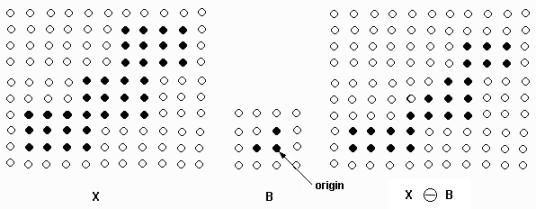对灰度图像的腐蚀：
如下图，左边是要处理图像，中间是结构元素，右边是与对应每个像素的灰度值。
处理过程就是：与上面的B一样，中间是要处理的元素所在的位置，三个1所在的位置对应三个灰度值，然后将中间这个1对应的灰度值改成这三个最小的，如源图像第一个灰度值1，它上左都没有灰度值，所以最小就是它本身，所以输出也是1，再比如处理灰度值为22那个点的时候，上面是7左边是44，所以22应改为7。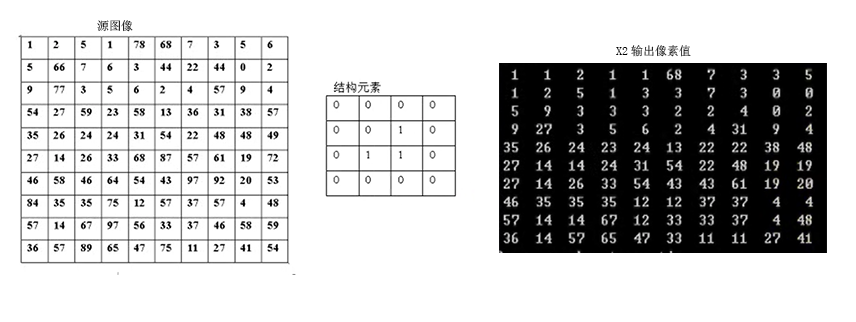6、OCR识别提取图片中文字原理
·  预处理：对包含文字的图像进行处理以便后续进行特征提取、学习。这个过程的主要目的是减少图像中的无用信息，以便方便后面的处理。在这个步骤通常有：灰度化（如果是彩色图像）、降噪、二值化、字符切分以及归一化这些子步骤。经过二值化后，图像只剩下两种颜色，即黑和白，其中一个是图像背景，另一个颜色就是要识别的文字了。降噪在这个阶段非常重要，降噪算法的好坏对特征提取的影响很大。字符切分则是将图像中的文字分割成单个文字——识别的时候是一个字一个字识别的。如果文字行有倾斜的话往往还要进行倾斜校正。归一化则是将单个的文字图像规整到同样的尺寸，在同一个规格下，才能应用统一的算法。

·  特征提取和降维：特征是用来识别文字的关键信息，每个不同的文字都能通过特征来和其他文字进行区分。对于数字和英文字母来说，这个特征提取是比较容易的，因为数字只有10个，英文字母只有52个，都是小字符集。对于汉字来说，特征提取比较困难，因为首先汉字是大字符集，国标中光是最常用的第一级汉字就有3755个；第二个汉字结构复杂，形近字多。在确定了使用何种特征后，视情况而定，还有可能要进行特征降维，这种情况就是如果特征的维数太高（特征一般用一个向量表示，维数即该向量的分量数），分类器的效率会受到很大的影响，为了提高识别速率，往往就要进行降维，这个过程也很重要，既要降低维数吧，又得使得减少维数后的特征向量还保留了足够的信息量（以区分不同的文字）。

· 分类器设计、训练和实际识别：分类器是用来进行识别的，就是对于第二步，对一个文字图像，提取出特征给，丢给分类器，分类器就对其进行分类，告诉你这个特征该识别成哪个文字。

·  后处理：后处理是用来对分类结果进行优化的，第一个，分类器的分类有时候不一定是完全正确的（实际上也做不到完全正确），比如对汉字的识别，由于汉字中形近字的存在，很容易将一个字识别成其形近字。后处理中可以去解决这个问题，比如通过语言模型来进行校正——如果分类器将“在哪里”识别成“存哪里”，通过语言模型会发现“存哪里”是错误的，然后进行校正。第二个，OCR的识别图像往往是有大量文字的，而且这些文字存在排版、字体大小等复杂情况，后处理中可以尝试去对识别结果进行格式化，比如按照图像中的排版排列什么的，举个栗子，一张图像，其左半部分的文字和右半部分的文字毫无关系，而在字符切分过程中，往往是按行切分的，那么识别结果中左半部分的第一行后面会跟着右半部分的第一行诸如此类。

代码：

TestOcr类：

package com.njupt.yangmaohu;

import java.io.File;
import java.io.IOException;

public class TestOcr {

public static void main(String[] args) {
// TODO 自动生成的方法存根
//输入图片地址
String path = "G:/ka.jpg";
PictureManage pictureManage = new PictureManage(path); //对图片进行处理
pictureManage.imshow();
try {
String valCode = new OCR().recognizeText(new File("xintu.jpg"), "jpg");//jpg是图片格式
System.out.println("图片中文字为："+"\n"+valCode);
} catch (IOException e) {
e.printStackTrace();
} catch (Exception e) {
e.printStackTrace();
}
}

}

PictureManage类：

package com.njupt.yangmaohu;

import java.awt.Graphics;
import java.awt.Image;
import java.awt.image.BufferedImage;
import java.awt.image.DataBufferByte;
import java.io.File;
import java.io.IOException;

import javax.imageio.ImageIO;
import org.opencv.core.Core;
import org.opencv.core.CvType;
import org.opencv.core.Mat;
import org.opencv.core.Size;
import org.opencv.highgui.Highgui;
import org.opencv.imgproc.Imgproc;

public class PictureManage {
private Mat image;
//private JLabel jLabelImage;
public PictureManage(String fileName) {
}

/**
* 图片画质处理
* @param image
* @return
*/
public static Mat setMatImage(Mat image) {
//灰度处理
Imgproc.cvtColor(image,image,Imgproc.COLOR_RGB2GRAY);
//二值化处理
Mat binaryMat = new Mat(image.height(), image.width(), CvType.CV_8UC1);
Imgproc.threshold(image, binaryMat,20, 300, Imgproc.THRESH_BINARY);

//图像腐蚀
Mat element = Imgproc.getStructuringElement(Imgproc.MORPH_RECT,
new Size(500,500));
Imgproc.erode(binaryMat, image,element);
}

/**
* Mat转image
* @param matrix
* @return
*/
private Image toBufferedImage(Mat matrix) {
int type = BufferedImage.TYPE_BYTE_GRAY;
if (matrix.channels()>1) {
type = BufferedImage.TYPE_3BYTE_BGR;
}
int bufferSize = matrix.channels()*matrix.cols()*matrix.rows();
byte[] buffer = new byte[bufferSize];
matrix.get(0, 0, buffer);
BufferedImage image = new BufferedImage(matrix.cols(), matrix.rows(),type);
final byte[] targetPxiels = ((DataBufferByte)image.getRaster().getDataBuffer()).getData();
System.arraycopy(buffer, 0, targetPxiels, 0, buffer.length);
return image;
}

/***
* 将Image变量保存成图片
* @param im
* @param fileName
*/
public  void  saveImage(Image im ,String  fileName) {
int w = im.getWidth(null);
int h = im.getHeight(null);
BufferedImage bi = new BufferedImage(w, h, BufferedImage.TYPE_3BYTE_BGR);
Graphics g = bi.getGraphics();
g.drawImage(im, 0, 0, null);
try {
ImageIO.write(bi, "jpg", new File(fileName));
} catch (IOException e) {
e.printStackTrace();
}
}

/**
* 图片处理
* @param args
*/
public void  imshow(){

//添加原图
Image originalImage = toBufferedImage(image);
saveImage(originalImage, "yuantu.jpg");
//jLabelImage.setIcon(new ImageIcon(originalImage));
//添加处理图
Mat mat1 = setMatImage(image);
Image newImage = toBufferedImage(mat1);
saveImage(newImage, "xintu.jpg");
}

}


ImageIOHelper类：

package com.njupt.yangmaohu;

import java.awt.image.BufferedImage;
import java.io.File;
import java.io.IOException;
import java.util.Iterator;
import java.util.Locale;

import javax.imageio.IIOImage;
import javax.imageio.ImageIO;
import javax.imageio.ImageWriteParam;
import javax.imageio.ImageWriter;
import javax.imageio.stream.ImageInputStream;
import javax.imageio.stream.ImageOutputStream;

import com.sun.media.imageio.plugins.tiff.TIFFImageWriteParam;

public class ImageIOHelper {
/**
* 图片文件转换为tif格式
* @param imageFile 文件路径
* @param imageFormat 文件扩展名
* @return
*/
public static File createImage(File imageFile, String imageFormat) {
File tempFile = null;
try {

ImageInputStream iis = ImageIO.createImageInputStream(imageFile);

//Set up the writeParam
TIFFImageWriteParam tiffWriteParam = new TIFFImageWriteParam(Locale.CHINESE);
tiffWriteParam.setCompressionMode(ImageWriteParam.MODE_DISABLED);

//Get tif writer and set output to file
Iterator<ImageWriter> writers = ImageIO.getImageWritersByFormatName("tiff");
ImageWriter writer = writers.next();

tempFile = tempImageFile(imageFile);
ImageOutputStream ios = ImageIO.createImageOutputStream(tempFile);
writer.setOutput(ios);
ios.close();

writer.dispose();

} catch (IOException e) {
e.printStackTrace();
}
return tempFile;
}

private static File tempImageFile(File imageFile) {
String path = imageFile.getPath();
StringBuffer strB = new StringBuffer(path);
strB.insert(path.lastIndexOf('.'),0);
return new File(strB.toString().replaceFirst("(?<=//.)(//w+)\$", "tif"));
}
}


OCR类：
package com.njupt.yangmaohu;

import java.io.File;
import java.io.FileInputStream;
import java.util.ArrayList;
import java.util.List;

import org.jdesktop.swingx.util.OS;

public class OCR {
private final String LANG_OPTION = "-l";  //英文字母小写l，并非数字1
private final String EOL = System.getProperty("line.separator");
private String tessPath = "C://Program Files (x86)//Tesseract-OCR";//tesseract-ocr安装地址
//private String tessPath = new File("tesseract").getAbsolutePath();

public String recognizeText(File imageFile,String imageFormat)throws Exception{
File tempImage = ImageIOHelper.createImage(imageFile,imageFormat);
File outputFile = new File(imageFile.getParentFile(),"output");
StringBuffer strB = new StringBuffer();
List<String> cmd = new ArrayList<String>();
if(OS.isWindowsXP()){
}else if(OS.isLinux()){
}else{
}

ProcessBuilder pb = new ProcessBuilder();
pb.directory(imageFile.getParentFile());

cmd.set(1, tempImage.getName());
pb.command(cmd);
pb.redirectErrorStream(true);

Process process = pb.start();
//tesseract.exe 1.jpg 1 -l chi_sim
int w = process.waitFor();

//删除临时正在工作文件
tempImage.delete();

if(w==0){

String str;
strB.append(str).append(EOL);
}
in.close();
}else{
String msg;
switch(w){
case 1:
msg = "Errors accessing files.There may be spaces in your image's filename.";
break;
case 29:
msg = "Cannot recongnize the image or its selected region.";
break;
case 31:
msg = "Unsupported image format.";
break;
default:
msg = "Errors occurred.";
}
tempImage.delete();
//throw new RuntimeException(msg);
}
new File(outputFile.getAbsolutePath()+".txt").delete();
return strB.toString();
}
}  

展开全文Java OpenCV 图像处理 字符识别
• OCR技术系列 身份证识别 深度学习实践系列之--身份证上汉字及数字识别系统的实现 https://github.com/Raymondhhh90/idcardocr:web部署,第二代居民身份证信息识别，速度略慢，待优化 https://github.co...
• OCR身份证识别的调研。典型身份证识别算法流程简介。图像处理 算法
• 图像处理开发资料、图像处理开发需求、图像处理接私活挣零花钱，可以搜索公众号"qxsf321"，并关注！ 图像处理开发资料、图像处理开发需求、图像处理接私活挣零花钱，可以搜索公众号"qxsf321"，...
• ocr识别中的图像分割方法小结 ...  ocr 字符图像的特点是：背景复杂，存在如底纹、水印、底线、框线、加盖印章干扰叠加 ,同时存在 光照...故图像的干扰和噪声大，采用普通的二值化分割方法效果不佳，故针对 OCR图像二值化 图像分割
• ## OCR技术原理

千次阅读 2019-11-21 11:17:36
OCR技术原理 文档识别技术功能特点图像输入：读取不同图像格式文件的算法。 图像预处理：主要包括图像二值化，噪声去除，倾斜较正等算法。 版面分析：将文档图片分段落，分行的算法就叫版面分析算法。 字符切割：...
• 最近想到做个文字识别的模块，其实文字识别例如车牌识别已经非常成熟的技术，学过图像处理的自己都可以写个算法来处理图像，不过懒人也有懒办法，一是用第三方专业做OCR的sdk，比如Tesseract和Asprise，其中前者用...图像处理
• Atalasoft成立于2000年，是一家来自美国马萨诸塞州的软件公司，其致力于为全球的软件开发公司及开发者提供优秀的商用图像处理软件及开发应用控件。他们的众多客户都是来自"财富"500强的图形软件开发公司，图形引擎...
• 获取图像红色通道（字体一般是黑色的，去除印章等其它因素） 二值化 邻域像素算法: 对于像素值&gt;245的邻域像素，判别为属于背景色，如果一个像素上下左右4各像素值有超过2个像素属于背景色，那么该像素就是...
• 前言 前段时间，项目中的一个核心模块中需要中文OCR功能，模型架构可以参考我的上一篇文章：AI实战：用DenseNet + CTC搭建中文OCR模型...图像处理（PIL、opencv） 流程图 重要说明 文本语料 语料取决于OC...
• 图像处理到底靠不靠谱做检测识别需不需要做图像预处理 图像识别中最核心最难搞的模块之一目标检测定位分割 图像识别 实际应用中的逻辑问题修复我们都知道单纯靠算法解决一切问题是不现实，图像识别作为一个工程性很...图像处理 算法
• opencv自带一个字符识别的例子，它的重点不是OCR字符识别，而主要是演示机器学习的应用。它应用的是UCI提供的字符数据（特征数据）。 DAMILES在网上发布了一个应用OpenCV进行OCR的例子， ...vector
• 图像处理开发资料、图像处理开发需求、图像处理接私活挣零花钱，可以搜索公众号"qxsf321"，并关注！ 图像处理开发资料、图像处理开发需求、图像处理接私活挣零花钱，可以搜索公众号"qxsf321"，...Tesseract
• ## python人工智能-图像识别

万次阅读 多人点赞 2019-08-03 16:43:15
PIL：（Python Imaging Library）是Python平台上的图像处理标准库，功能非常强大。 pytesseract：图像识别库。 我这里使用的是python3.6，PIL不支持python3所以使用如下命令 pip install pytesseract pip ...图像识别 python PIL
• ## Python+OpenCV+pytesseract 识别 银行卡号

万次阅读 热门讨论 2017-12-13 17:00:09
首先给大家看下什么是OCR-A字体： 　 　尽管现代OCR系统不需要专门的字体（如OCR-A），但仍被广泛应用于身份证，报表和信用卡。 ...　1....　为了实现这一点，我们需要应用一些图像处理操作，包
• ## OCR识别的开源分析

万次阅读 多人点赞 2016-06-08 16:36:45
OCR识别的开源分析OCR软件主要是由下面几个部分组成。　1. 图像输入、预处理： 　2. 图像输入：对于不同的图像格式，有着不同的存储格式，不同的压缩方式。预处理：主要包括二值化，噪声去除，倾斜较正等 　3. 二...深度学习 图像处理 机器学习
• ## OCR 识别原理

千次阅读 2018-10-09 11:02:30
拍照怎么搜题？（上） ...amp;mid=402907292&amp;idx=1&amp;sn=889c4abcf576e24525ea6a705069c4de ...1、图像处理。分别是：  a、灰度处理：将彩色图片变成灰度图  b、二值化：将灰度图变成黑白图  ...ocr原理
...